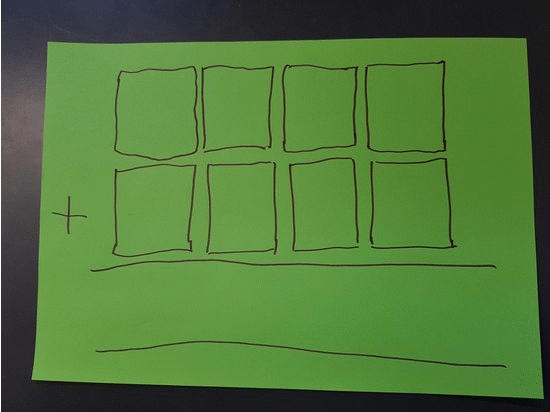#### You may also like### Adding All Nine

Make a set of numbers that use all the digits from 1 to 9, once and once only. Add them up. The result is divisible by 9. Add each of the digits in the new number. What is their sum? Now try some other possibilities for yourself!### Counting Factors

Is there an efficient way to work out how many factors a large number has?### Repeaters

Choose any 3 digits and make a 6 digit number by repeating the 3 digits in the same order (e.g. 594594). Explain why whatever digits you choose the number will always be divisible by 7, 11 and 13.

# Legs Eleven

##### Age 11 to 14Challenge Level

Take any four-digit number.
Create a second number by moving the first digit to the 'back of the queue' and moving the rest along.
I predict your answer will be a multiple of 11...Try it a few times. Is the answer always a multiple of $11$?
Can you explain why?

Click below to see what Samira noticed:

"I started with 5 thousands, 2 hundreds, 3 tens and 8 units.
After I moved the digits along, my new number had 2 thousands, 3 hundreds, 8 tens and 5 units.

I wonder if this can help me explain what's happening?"

Click below to see what Jay noticed:

"I picked 1000 as my first number, so my second number was 0001 and the total was 1001.
I know 1001 is a multiple of 11 because it is 1100-99, and 1100 and 99 are both multiples of 11."

Do these observations help you to explain what's going on?

What if you start with a three-digit number?
Or a five-digit number?
Or a six-digit number?
Or a 38-digit numbers ... ?

Can you prove your findings?

You may be interested in this article on Divisibility Tests.

Click here for a poster of this problem.